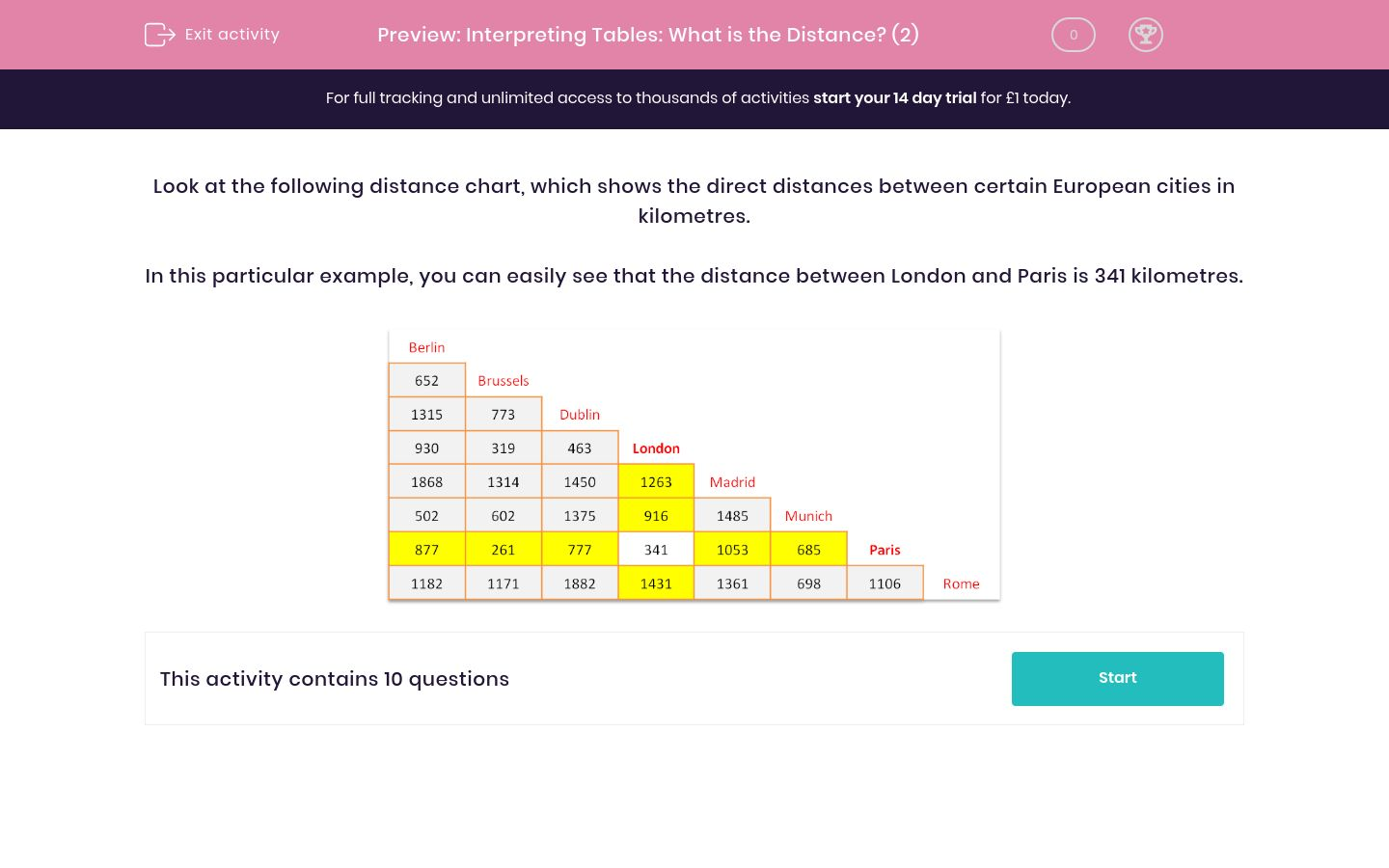# Interpreting Tables: What is the Distance? (2)

In this worksheet, students read a distance chart then answer questions on the distances between two cities.Key stage:  KS 2

Curriculum topic:   Maths and Numerical Reasoning

Curriculum subtopic:   Interpreting Data

Difficulty level:### QUESTION 1 of 10

Look at the following distance chart, which shows the direct distances between certain European cities in kilometres.

In this particular example, you can easily see that the distance between London and Paris is 341 kilometres.Use this distance chart to state the distance in km between the following two cities:

Just write the number.Use this distance chart to state the distance in km between the following two cities:

Munich and London

Just write the number.Use this distance chart to state the distance in km between the following two cities:

Berlin and Dublin

Just write the number.Use this distance chart to state the distance in km between the following two cities:

London and Rome

Just write the number.Use this distance chart to state the distance in km between the following two cities:

Just write the number.Use this distance chart to state the distance in km between the following two cities:

Paris and Rome

Just write the number.Use this distance chart to find which city is furthest away from Rome.Use this distance chart to find which of Berlin or Brussels is closer to Munich?Use this distance chart to find how much closer to Paris, London is compared to Rome?

Just write the number.Which two cities are closest together?Brussels and Paris

• Question 1

Use this distance chart to state the distance in km between the following two cities:

Just write the number.1868
• Question 2

Use this distance chart to state the distance in km between the following two cities:

Munich and London

Just write the number.916
• Question 3

Use this distance chart to state the distance in km between the following two cities:

Berlin and Dublin

Just write the number.1315
• Question 4

Use this distance chart to state the distance in km between the following two cities:

London and Rome

Just write the number.1431
• Question 5

Use this distance chart to state the distance in km between the following two cities:

Just write the number.1485
• Question 6

Use this distance chart to state the distance in km between the following two cities:

Paris and Rome

Just write the number.1106
• Question 7

Use this distance chart to find which city is furthest away from Rome.Dublin
EDDIE SAYS
Furthest city from Rome is Dublin.
• Question 8

Use this distance chart to find which of Berlin or Brussels is closer to Munich?Berlin
• Question 9

Use this distance chart to find how much closer to Paris, London is compared to Rome?

Just write the number.765
EDDIE SAYS
Paris and Rome: 1106 Km. Paris and London: 341 Km.
• Question 10

Which two cities are closest together?Brussels and Paris
EDDIE SAYS
Brussels and Paris are the two cities closest together (261 Km).
---- OR ----

Sign up for a £1 trial so you can track and measure your child's progress on this activity.

### What is EdPlace?

We're your National Curriculum aligned online education content provider helping each child succeed in English, maths and science from year 1 to GCSE. With an EdPlace account you’ll be able to track and measure progress, helping each child achieve their best. We build confidence and attainment by personalising each child’s learning at a level that suits them.

Get started